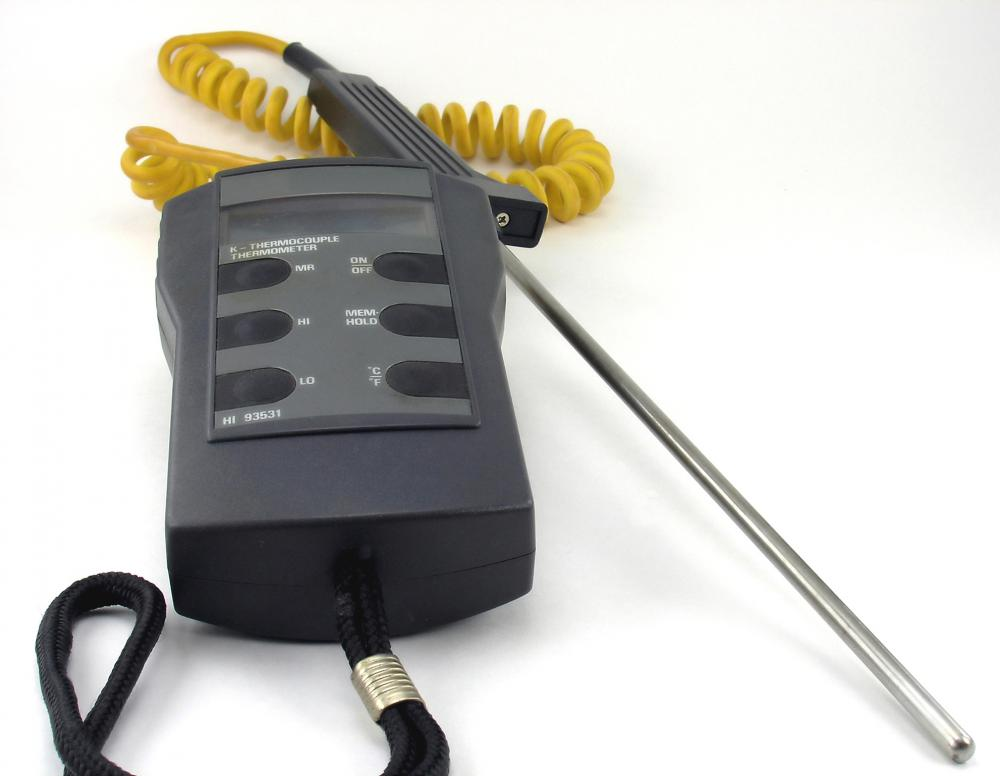# What is the Seebeck Effect?

Christopher Fox Graham

The Seebeck Effect describes a thermoelectric phenomenon by which temperature differences between two dissimilar metals in a circuit converts into an electric current.Thermocouple thermometers are tools based on measuring the Seebeck Effect and the Peltier and Thompson effects.

Discovered in 1821, the Seebeck Effect is one of three reversible phenomena describing similar processes relating to thermoelectricity, conductivity and temperature. The Peltier Effect was first observed in 1834 and the Thomson Effect was first explained in 1851.Thomas Johann Seebeck utilized a compass during his experiments relating to metals and electricity.

The Seebeck Effect is named for East Prussian scientist Thomas Johann Seebeck (1770-1831). In 1821, Seebeck discovered that a circuit made of two dissimilar metals conducts electricity if the two places where the metals connect are held at different temperatures. Seebeck placed a compass near the circuit he built and noticed that the needle deflected. He discovered that the deflection’s magnitude increased proportionally as the temperature difference increased. His experiments also noted that the temperature distribution along the metal conductors did not affect the compass. However, changing the types of metals he used did change the magnitude that the needle deflected.

The Seebeck Coefficient is a number describing the voltage produced between two points on a conductor, where a uniform temperature difference of 1 kelvin exists between the points. The metals in Seebeck’s experiments were reacting to the temperatures, creating a current loop in the circuit and a magnetic field. Unaware of an electric current at the time, Seebeck erroneously assumed this was a thermomagnetic effect.

In 1834, French scientist Jean Charles Athanase Peltier (1784-1845) described the second closely related phenomena, now known as the Peltier Effect. In his experiment, Peltier changed the voltage between the metal conductors and discovered that the temperature at either junction changed proportionally. In 1839, German scientist Heinrich Lenz (1804-1865) expanded on Peltier’s discovery and described heat transfer at the junctions, depending on the direction that the current flows along the circuit. While these two experiments were focused on different parts of the circuit and the thermoelectric effects, they are often referred to simply as the Seebeck-Peltier Effect or the Peltier-Seebeck Effect.

In 1851, British physicist William Thomson (1824-1907), later known as the first Baron Kelvin, observed that the heating or cooling of a single type of metal conductor from an electrical current. The Thomson Effect describes the rate of heat created or absorbed in a current-carrying metal or other conductive material, subjected to a temperature gradient.

Thermocouple thermometers are electrical engineering tools based on measuring the Seebeck Effect and the Peltier and Thompson effects. The thermometers work by converting the thermal potential difference into electric potential difference.

## You might also Likeanon989433

It is perfectly correct to say "1 degree Celcius". Kelvin is different because it is a hypothetical temperature. By convention, one simply says "1 kelvin" or "3 kelvins." We can also use a capital "K" for kelvin.

anon143887

this is for my physics homework, but let's say they give me the voltage produced. how can i find the values for the temperature differences?

anon129944

this helped me with my science fair topic.